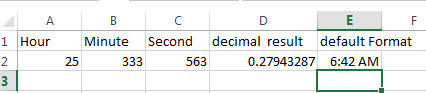# Excel TIME Function

This post will guide you how to use Excel TIME function with syntax and examples in Microsoft excel.

### Description

The Excel TIME function returns a date in serial number format for a particular time (the hour, minute and second).

The serial number returned by TIME function is a value between 0 and 0.99988426. And the result of 0 represents 12:00:00 AM and the result of 0.99988426 represents 11:59:59 PM.

So this function can be used to create a time with hours, minutes, and seconds. And it also will return a decimal number representing a particular time in excel.

The TIME function is a build-in function in Microsoft Excel and it is categorized as a DATE and TIME Function.
The TIME function is available in Excel 2016, Excel 2013, Excel 2010, Excel 2007, Excel 2003, Excel XP, Excel 2000, Excel 2011 for Mac.

### Syntax

The syntax of the TIME function is as below:

`= TIME(hour, minute, second)`

Where the TIME function arguments are:

• Hour -This is a required argument. A number from 0 (zero) to 32767 representing the hour. If the value is greater than 23 and then it will be divided by 24 and the remainder will be treated as the hour value. For example, the TIME(28,0,0) is equal to TIME(4,0,0).
• Minute – This is a required argument. A number from 0 to 32767 representing the minute. If the minute value is greater than 59 and it will be converted to hours and minutes (it means that every 60 minutes will add one hour to hour value). For example, TIME(1,75,0) = TIME(2,15,0).
• Second – This is a required argument. A number from 0 to 32767 representing the second. If the second value is greater than 59 and it will be converted to hours, minutes, and seconds (it means that every 60 seconds will add 1 minute to minute value). For example, TIME(1,20,75) = TIME(1,21,15) .

Excel TIME Function Examples
The below examples will show you how to use Excel TIME function to create a decimal number representing a particular time.

#1 =TIME(A2,B2,C2)